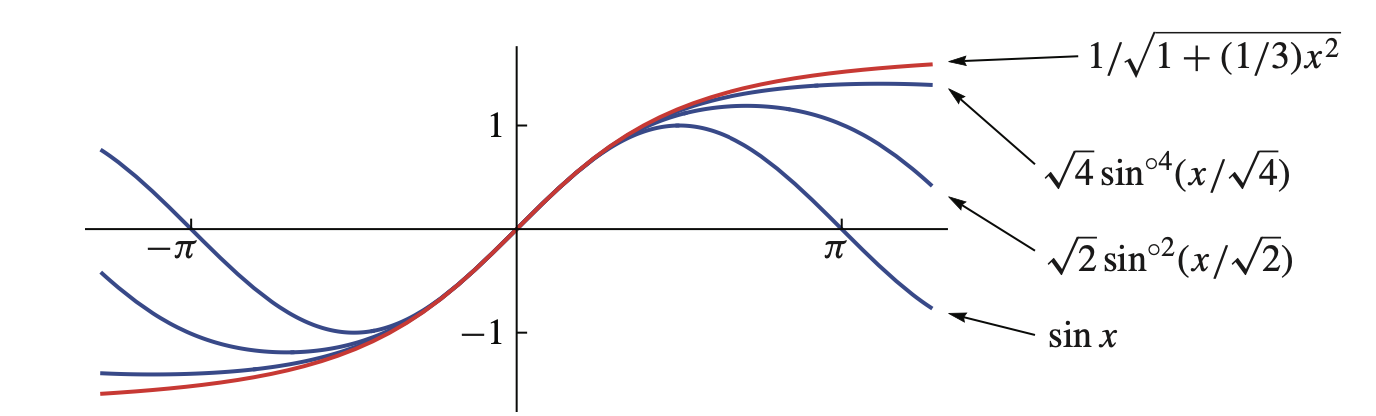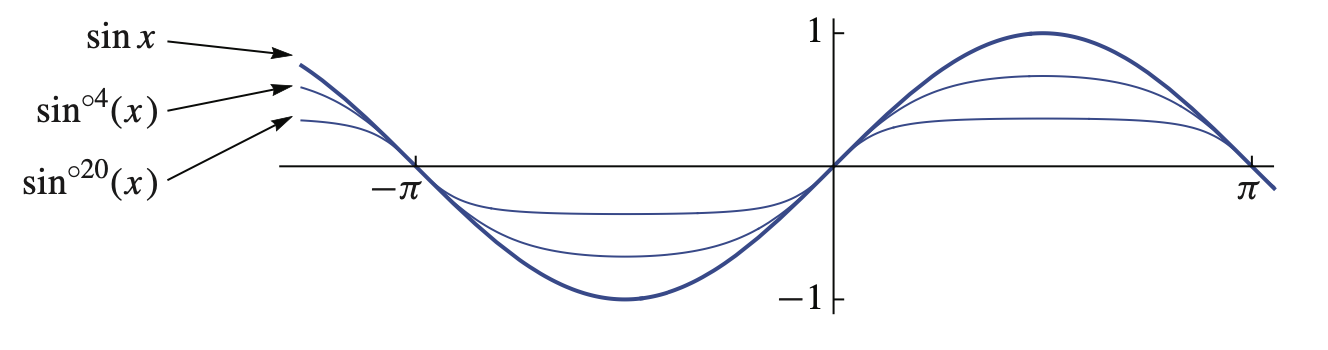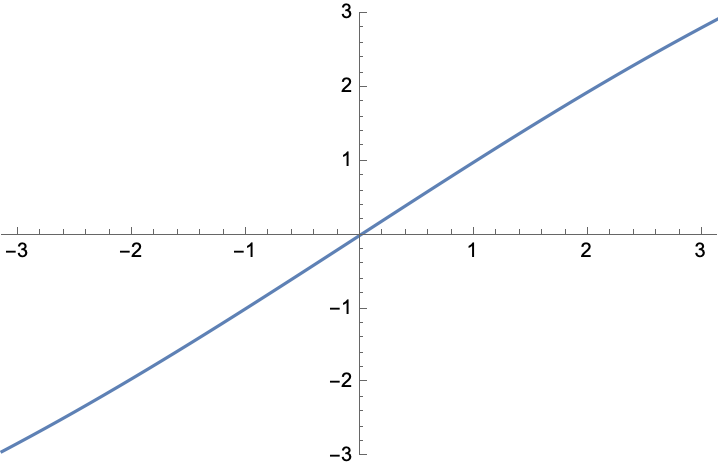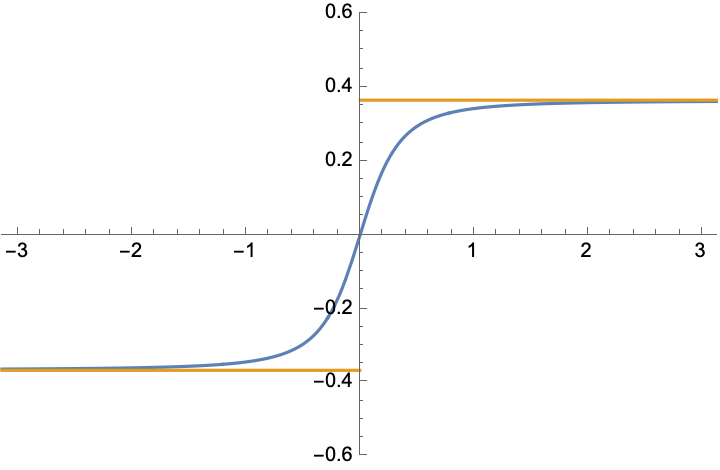# Behavior of $n^\alpha \sin^{\circ\, n}(n^{-\alpha}x)$

I'll write it formally: Let $$\sin^{\circ\, 1}(x) = \sin(x)$$ and $$\sin^{\circ n+1}(x) = \sin\bigl(\sin^{\circ n}(x)\bigr)$$ for $$n\in \Bbb N$$ with $$n>1$$. What is the limit as $$n \to \infty$$?

It's fairly easy to prove that the absolute value of $$f(n,x)$$ is decreasing for every constant $$x$$ as $$n$$ is increasing, so the limit exists. From what I calculated, it seems like $$f(\infty,x)= 0$$ for all $$x$$. Does someone have a proof for that?

Follow up: The limit $$\sin^{\circ n}(x)\rightarrow 0$$ may be a bit trivial. What if we rescale $$x$$ and ask for the limit of $$n^\alpha \sin^{\circ n}(n^{-\alpha}x)$$ as $$n\rightarrow\infty$$? For which $$\alpha$$ does the limit exist and what is it?

• If the limit exists it must be a fixed point if the sine. Oct 2 at 19:24
• And the limit exists since $\sin(x) \in [-1,1]$ and on $[-1,1]$ you have that $\sin x$ and $x$ have the same sign, while $|\sin x| \leq x$. So the generated sequence is eventually monotone. Oct 2 at 19:48
• to make this question less trivial and more appropriate for MO, I have added the rescaled limit as a follow-up question; perhaps if it's reopened the limit for $\alpha\neq 0,1/2$ can be considered in an answer. Oct 3 at 6:56
• See math.stackexchange.com/questions/3837335/… for the square root case and links to other topics related to the sine iteration. Oct 3 at 16:37
• cosine converges at non-zero Oct 3 at 18:18

A rescaling is needed for a nontrivial limit. As discussed in Iteration of Sine and Related Power Series by C. Towse (2014), denoting the $$n$$-th iterate by $$\sin^{\circ n}x$$, one has the limit $$\lim_{n\rightarrow\infty}\sqrt n\sin^{\circ n}(x/\sqrt n)=\frac{x}{\sqrt{1+x^2/3}}.$$ The graph (from the cited paper) shows that the limit is attained quite rapidly.Without the rescaling the iterated sine converges to zero (for the reasons indicated in the comments to the OP). The convergence is slow, see the graph.For the general rescaling, $$z_\alpha(x)=\lim_{n\rightarrow\infty}n^\alpha\sin^{\circ n}(n^{-\alpha}x),$$ I surmise (based on the small-$$x$$ expansion of the sine$$^\ast$$) that the limit is $$z_\alpha(x)=0$$ for $$\alpha<1/2$$ and $$z_\alpha(x)=x$$ for $$\alpha>1/2$$. This agrees quite well with the numerics, see plots belowPlots of $$n^\alpha\sin^{\circ n}(n^{-\alpha}x)$$ for $$n=500$$ and $$\alpha=0.75$$ (left) and $$\alpha=0.25$$ (right). The horizontal lines in the right plot show the asymptote $$(\text{sign}\,x)\,\sqrt{3}\,n^{\alpha-1/2}$$.

$$^\ast$$ For $$\alpha<1/2$$ we can proceed as follows: Assume a power law decay, $$y_n=(\text{sign}\,x)\,n^\alpha\sin^{\circ n}(n^{-\alpha}x)=(\text{sign}\,x)cn^{-p}$$, substitute into $$y_{n+1}=(n+1)^\alpha \sin(y_n/n^\alpha)$$, and expand for large $$n$$. So we have $$c(n+1)^{-p}\approx cn^{-p}-cpn^{-p-1},\;\; (n+1)^\alpha\sin(cn^{-p-\alpha})\approx cn^{-p}+\alpha cn^{-p-1}-\tfrac{1}{6}c^3n^{-3p-2\alpha},$$ and equating these two expressions gives $$p=\tfrac{1}{2}-\alpha$$, $$c=\sqrt{6(p+\alpha)}=\sqrt{3}$$. We thus arrive at the large-$$n$$ asymptotics $$n^\alpha\sin^{\circ n}(n^{-\alpha}x)\rightarrow (\text{sign}\,x)\,\sqrt{3}\,n^{\alpha-1/2}\rightarrow 0,\;\;\text{for}\;\;\alpha<1/2.$$

• At first I had some misgivings about one changing a question and then answering it, but looking up the paper I can see that in this case it is both justified and interesting. Oct 3 at 12:48
• $$x-\frac{x^3}{6}<\sin(x)<x$$ for $x>0$, so $$\sin^{ck}(x)-\frac16 (\sin^{ck}(x))^3 < \sin^{c(k+1)}(x) < \sin^{ck}(x),$$ which in turn yields $$\sin^{ck}(x)-\frac{x^3}{6}<\sin^{c(k+)1}(x)<x, \textrm{ and } x-n\frac{x^3}{6}<\sin^{cn}(x)<x$$ So writing $v_n= n^\alpha \sin^{cn}\left(n^{-\alpha}x\right)$, we have $$x- n^{1-2\alpha} \frac{x^3}{6} < v_n(x) < x$$ So when $\alpha > \frac12$, $v_n\to x$. Oct 3 at 18:24
• @Yaakov, you were not the only one with misgivings. See meta.mathoverflow.net/questions/5150/… where the question Oct 4 at 2:23